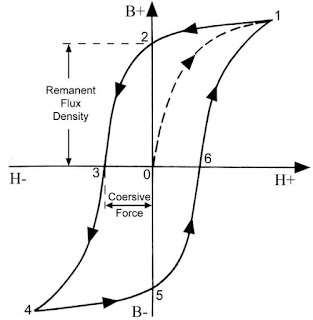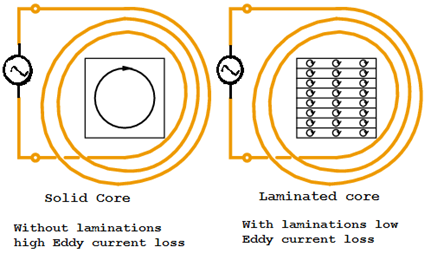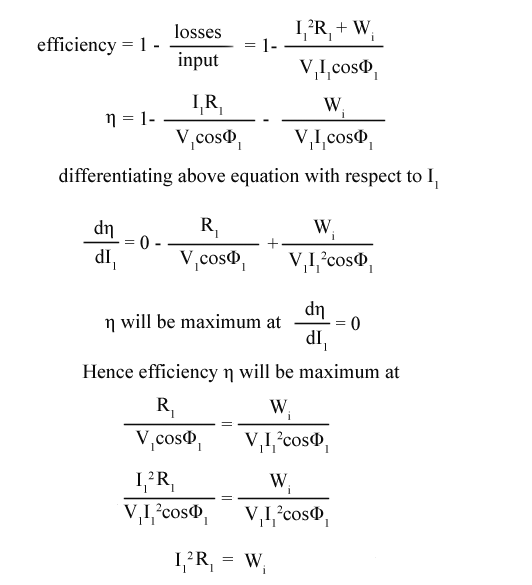# Losses In Transformers ; Hysteresis Loss, Eddy Current Loss, Efficiency

We have discussed electrical transformer construction, working When it comes to efficiency of the transformer it is above 95%.In modern power transformers, we can get above 98% of efficiency. An electrical transformer is a static device, so no rotating losses or frictional, windage losses.So no mechanical losses occur in transformer.We consider only electrical losses in the transformer.Now read efficiency of transformer and losses in transformer below.### Losses in Transformer

As we no machine in this world is ideal.The transformer is also not an exception to this. There are two types of losses in transformers they are
(i) Core Losses Or Iron Losses
(ii) Copper Loss In Transformer

### (i) Core Losses Or Iron Losses in Transformer

Eddy current, Hysteresis losses are considered as core losses of transformer.Core losses of transformer almost constant for a transformer after it is built for certain and frequency.Because eddy current loss and hysteresis loss depends on the magnetic properties, volume of the core which is used for the construction.As volume is fixed we can say core losses or iron losses strictly depends only on frequency.

Hysteresis Loss in transformer

Hysteresis losses occur due to reversal of magnetization in the transformer core.The magnetizing and demonstration curve of any material will not be same.Some loss happens due to cohesive force between magnetic atoms.The hysteresis loss in transformer depends on the volume and grade of the iron, frequency of magnetic reversals and value of flux density. It can be given by, Steinmetz formula:

Wh= ηBmax1.6fV (watts)

where,   η = Steinmetz hysteresis constant
V = volume of the core in m3

#### How to reduce Hysteresis Loss in Transformer?

We can reduce hysteresis losses by using Cold Rolled Grain Oriented (CRGO) Silicon Steel.In earlier days we used silicon steel as transformer core after that CRGO steel found less hysteresis constant.
Eddy current loss in transformer
The transformer works only on alternating current when AC current is supplied to the primary winding of transformer, it sets up alternating magnetizing flux. When this flux cuts the secondary winding, emf will be induced in it.This main flux also cuts the core of the transformer, As we know whenever flux cuts the magnetic material emf will induce in it.which will result in small circulating currents in them due to closed path of the core. This induced current is called as eddy current. Due to eddy currents, some energy will be dissipated in the form of heat.
We= ηB²f²T (watts)
We ∝ T
where,   η = Steinmetz hysteresis constant
T = Thickness of lamination in m

How to reduce Eddy current loss in transformer?By making the core into laminations.In the above relation, you can find that eddy current losses are directly proportional with square of its thickness.As the lamination thickness is much smaller than the depth of penetration of the ﬁeld, the eddy current loss can be reduced by reducing the thickness of the lamination. Present day we use lamination thickness of 0.25mm operated at 2 Tesla.By decreasing lamination thickness we can reduce the eddy current losses in the core.This loss also remains constant until the frequency of operation is constant.

### (ii) Copper Loss In Transformer

Copper loss in transformer also known as ohmic losses.This loss due to resistance of copper winding.We know that when current flows through a conductor definitely there will be I²R loss in the form of heat.
1.Primary copper losses in transformer take place due to the flow of current in the primary winding of transformer.
2.Secondary copper losses take place due to the flow of current in the secondary winding of transformer.
Total copper losses=I²R=(I1)²R1+(I2)²R2
where, I1, I2 and R1, R2 are current and resistances of primary, secondary winding respectively.
The primary and secondary resistances diﬀer from their d.c. values due to skin eﬀect and the temperature rise of the winding.
Apart from these major losses we have dielectric loss in transformer.As its name says dielectric losses take place in the insulation coating of the transformer due to the large electric stress.It is constant and in the case of low rating transformers, it is neglected.

### Efficiency Of Transformer

Like all machines efficiency of a transformer can be defined as the output power divided by the input power.
Efficiency = output / input
As a transformer being highly efficient[95-99%], output and input are having nearly same value, So it is impractical to measure the efficiency of transformer by using output/input.A practical method to find efficiency of a transformer is using, efficiency = (input – losses) / input = 1 – (losses / input).
Condition For Maximum Efficiency
Let,
Copper loss = (I1)²R1
Iron loss = WiHence, efficiency of a transformer will be maximum when copper loss and iron losses are equal.That is Copper loss = Iron loss.